## C++与正则表达式

Posted on Mar 17, 2020# 代码示例

git clone https://github.com/paulQuei/cpp-regex.git


C++中正则表达式的API基本上都位于<regex>头文件中。

#include <iostream>
#include <regex>

using namespace std;


# 入门示例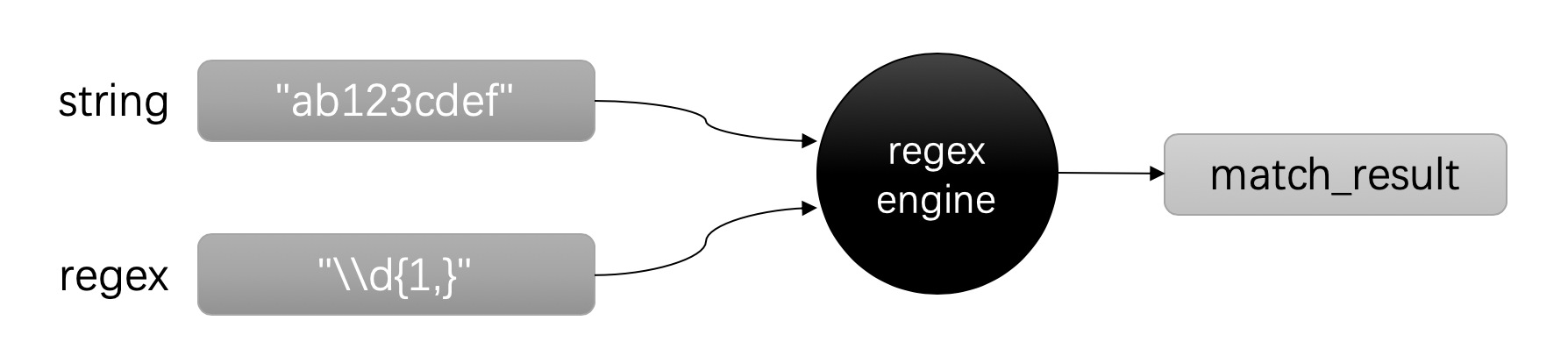## 匹配

string s1 = "ab123cdef"; // ①
string s2 = "123456789"; // ②

regex ex("\\d+"); // ③

cout << s1 << " is all digit: " << regex_match(s1, ex) << endl; // ④
cout << s2 << " is all digit: " << regex_match(s2, ex) << endl; // ⑤


1. 这是一个包含了数字和字母的字符串
2. 这是一个只包含了数字的字符串
3. 这是我们的正则表达式，它表示：有多个数字
4. 通过regex_match判断第一个字符串是否匹配，这里将返回false
5. 通过regex_match判断第二个字符串是否匹配，这里将返回true

ab123cdef is all digit: 0
123456789 is all digit: 1


## 搜索

string s = "ab123cdef"; // ①
regex ex("\\d+");    // ②

smatch match; // ③
regex_search(s, match, ex); // ④

cout << s << " contains digit: " << match << endl; // ⑤

1. 这是一个包含了数字和字母的字符串
2. 和前面一样的正则表达式
3. 通过std::smatch来保存匹配的结果。除了std::smatch，还有std::cmatch也很常用。前者是以std::string的形式返回结果，后者是以const char*的形式返回结果。
4. 通过regex_search函数搜索结果
5. 打印出匹配的结果

ab123cdef contains digit: 123


## 替换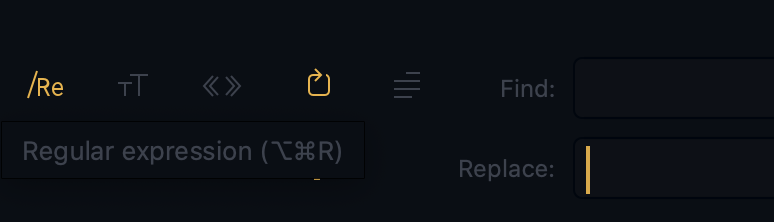string s = "ab123cdef"; // ①
regex ex("\\d+");    // ②

string r = regex_replace(s, ex, "xxx"); // ③

cout << r << endl; // ④

1. 仍然是前面这个字符串
2. 仍然是同样的正则表达式
3. 通过regex_replace完成替换
4. 通过cout输出结果

abxxxcdef


# 正则表达式文法

## 文法

C++中内置了多种正则表达式文法，在创建正则表达式的时候可以通过参数来选择。

ECMAScript ECMAScript正则表达式语法，默认选项
basic 基础POSIX正则表达式语法
extended 扩展POSIX正则表达式语法
awk awk工具的正则表达式语法
grep grep工具的正则表达式语法
egrep grep工具的正则表达式语法

grep的全称是Global Regular Expression Print。这个名字是在提示我们，它本身与正则表达式的历史有着特定的联系。

C++ 中的 ECMAScript 正则表达式文法是 ECMA-262 文法，你可以点击链接查看详细内容。

## Raw string literal

Raw string literal的格式如下：

R"delimiter(raw_characters)delimiter"


• delimiter是可选的分隔符，通常不用写
• raw_characters是具体的字符串

string s = R"("\w\\w\\\w)";
cout << s << endl;


"\w\\w\\\w


. 匹配任意字符
[ 字符类的开始
] 字符类的结束
{ 量词重复数开始
} 量词重复数结束
( 分组开始
) 分组结束
\ 转义字符
\\ 转义字符自身
* 量词，0个或者多个
+ 量词，1个或者多个
? 量词，0个或者1个
|
^ 行开始；否定
$行结束 \n 换行 \t Tab符 \xhh hh表示两位十六进展表示的Unicode字符 \xhhhh hhhh表示四位十六进制表示的Unicode字符串 这些字符并不少，刚开始接触可能记不住，但随着下文的讲解，相信你会逐渐熟悉它们。 ## 字符类 字符类，顾名思义：是对字符的分类。 例如：1234567890这些都属于数字字符类。除此之外，还有其他的分类，它们如下表所示： 字符类 简写 说明 [[:alnum:]] 字母和数字 [_[:alnum:]] \w 字母，数字以及下划线 [^_[:alnum:]] \W 非字母，数字以及下划线 [[:digit:]] \d 数字 [^[:digit:]] \D 非数字 [[:space:]] \s 空白字符 [^[:space:]] \S 非空白字符 [[:lower:]] 小写字母 [[:upper:]] 大写字母 [[:alpha:]] 任意字母 [[:blank:]] 非换行符的空白字符 [[:cntrl:]] 控制字符 [[:graph:]] 图形字符 [[:print:]] 可打印字符 [[:punct:]] 标点字符 [[:xdigit:]] 十六进制的数字字符 这里我们可以看到： • 字符类通过[]作为标识，因此这两个字符是正则表达式的中的特殊字符。如果是想使用这两个字符本身，需要对它们进行转义。 • []内部，通过[:xxx:]来描述字符类的名称。 • []中可以通过^表示否定，即：字符类的反面。 • 字母，数字和空白字符由于这些字符类非常常用，因此它们有简写的方法。简写使得正则表达式更加简洁，但表达的含义是一样的。 接下来我们看一个代码示例： #include <iostream> #include <regex> using namespace std; static void search_string(const string& str, const regex& reg_ex) { // ① for (string::size_type i = 0; i < str.size() - 1; i++) { auto substr = str.substr(i, 1); if (regex_match(substr, reg_ex)) { cout << substr; } } } static void search_by_regex(const char* regex_s, const string& s) { // ② regex reg_ex(regex_s); cout.width(12); // ③ cout << regex_s << ": \""; // ④ search_string(s, reg_ex); // ⑤ cout << "\"" << endl; } int main() { string s("_AaBbCcDdEeFfGg12345 \t\n!@#$%"); // ⑥

search_by_regex("[[:alnum:]]", s);          // ⑦
search_by_regex("\\w", s);                  // ⑧
search_by_regex(R"(\W)", s);                // ⑨
search_by_regex("[[:digit:]]", s);          // ⑩
search_by_regex("[^[:digit:]]", s);         // ⑪
search_by_regex("[[:space:]]", s);          // ⑫
search_by_regex("\\S", s);                  // ⑬
search_by_regex("[[:lower:]]", s);          // ⑭
search_by_regex("[[:upper:]]", s);
search_by_regex("[[:alpha:]]", s);          // ⑮
search_by_regex("[[:blank:]]", s);          // ⑯
search_by_regex("[[:graph:]]", s);          // ⑰
search_by_regex("[[:print:]]", s);          // ⑱
search_by_regex("[[:punct:]]", s);          // ⑲
search_by_regex("[[:xdigit:]]", s);         // ⑳

return 0;
}


1. 这里定义了一个函数，它接受一个字符串和一个正则表达式作为输入。该函数遍历字符串，每次取出一个字符然后用正则表达式进行匹配，如果匹配上，则输出该字符。逐个遍历字符串的方式并不是非常好，在后文中我们将看到更好的方法。
2. search_by_regex将调用search_string进行字符的匹配。
3. cout.width(12); 是为了控制输出格式的缩进。
4. 先打印出正则表达式，然后打印冒号和双引号。将匹配的内容放在双引号中是为了更容易辨识。
5. 调用search_string进行字符的匹配。
6. 这是我们待匹配的字符串，它其中包含了各种类型的字符。
7. [[:alnum:]]匹配字母和数字类字符。
8. \w[_[:alnum:]]的简写方式，它与字符数字的区别在与：它还包含了_。当通过字符串定义正则表达式时，反斜杠需要转义。
9. R"(\W)"是一个Raw string literal，因此，这里的反斜杠不再需要转义。
10. [[:digit:]]匹配数字类字符。
11. [^[:digit:]]是非数字类正则表达式，它与⑩正好相反。
12. [[:space:]]匹配空白类字符，该表达式将包含换行符。
13. \S是非空白类字符类。
14. [[:lower:]]小写字母，[[:upper:]]下一行是大写字母。
15. [[:alpha:]]匹配所有字母字符。
16. [[:blank:]]是空白字符类，它与[[:space:]]的区别是：它不包含换行符。
17. [[:graph:]]是图形类字符。
18. [[:print:]]是可打印字符。
19. [[:punct:]]是标点符号字符。
20. [[:xdigit:]]是十六进制的数字字符。

 [[:alnum:]]: "AaBbCcDdEeFfGg12345"
\w: "_AaBbCcDdEeFfGg12345"
\W: "
!@#$" [[:digit:]]: "12345" [^[:digit:]]: "_AaBbCcDdEeFfGg !@#$"
[[:space:]]: "
"
\S: "_AaBbCcDdEeFfGg12345!@#$" [[:lower:]]: "abcdefg" [[:upper:]]: "ABCDEFG" [[:alpha:]]: "AaBbCcDdEeFfGg" [[:blank:]]: " " [[:graph:]]: "_AaBbCcDdEeFfGg12345!@#$"
[[:print:]]: "_AaBbCcDdEeFfGg12345 !@#$" [[:punct:]]: "_!@#$"
[[:xdigit:]]: "AaBbCcDdEeFf12345"


## 重复

{n} 重复n次
{n,} 重复n或更多次
{n,m} 重复[n ~ m]次
* 重复0次或多次，等同于{0,}
+ 重复1次或多次，等同于{1,}
? 重复0次或1次，等同于{0,1}

#include <iostream>
#include <regex>

using namespace std;

static void search_by_regex(const char* regex_s,
const string& s) { // ①
regex reg_ex(regex_s);
smatch match_result; // ②
cout.width(14); // ③
if (regex_search(s, match_result, reg_ex)) { // ④
cout << regex_s << ": \"" << match_result << "\"" << endl; // ⑤
}
}

int main() {
string s("_AaBbCcDdEeFfGg12345!@#$% \t"); // ⑥ search_by_regex("[[:alnum:]]{5}", s); // ⑦ search_by_regex("\\w{5,}", s); // ⑧ search_by_regex(R"(\W{3,5})", s); // ⑨ search_by_regex("[[:digit:]]*", s); // ⑩ search_by_regex(".+", s); // ⑪ search_by_regex("[[:lower:]]?", s); // ⑫ return 0; }  在这段代码中： 1. 这里定义了一个函数，它接受一个正则表达式和字符串。 2. match_result用来存储查找的结果。 3. 设置输出格式，为了让输出对齐。 4. 通过regex_search在字符串中查找匹配字符。 5. 输出匹配的结果。 6. 待匹配的字符串。 7. [[:alnum:]]{5}是指：字符或者数字出现5次。 8. \\w{5,}是指：字母，数字或者下划线出现5次或更多次。 9. R"(\W{3,5})"是指：非字母，数字或者下划线出现3次到5次。 10. [[:digit:]]*是指：数字出现任意多次。 11. .+是指：任意字符出现至少1次。 12. [[:lower:]]?是指：小写字母出现0次或者1次。 该程序输出如下： [[:alnum:]]{5}: "AaBbC" \w{5,}: "_AaBbCcDdEeFfGg12345" \W{3,5}: "!@#$%"
[[:digit:]]*: ""
.+: "_AaBbCcDdEeFfGg12345!@#$% " [[:lower:]]?: ""  # 正则表达式编程 接下来我们会看到更多的示例。同时，也会看到C++正则表达式API的更多功能。 为了便于下文示例的讲解，我们以维基百科上对于正则表达式的介绍文本为基础。 A regular expression, regex or regexp$($sometimes called a rational expression$)$is a sequence of characters that define a search pattern. Usually such patterns are used by string searching algorithms for “find” or “find and replace” operations on strings, or for input validation. It is a technique developed in theoretical computer science and formal language theory. The concept arose in the 1950s when the American mathematician Stephen Cole Kleene formalized the description of a regular language. The concept came into common use with Unix text-processing utilities. Different syntaxes for writing regular expressions have existed since the 1980s, one being the POSIX standard and another, widely used, being the Perl syntax. Regular expressions are used in search engines, search and replace dialogs of word processors and text editors, in text processing utilities such as sed and AWK and in lexical analysis. Many programming languages provide regex capabilities either built-in or via libraries. 我们将这段文字保存在名称为content.txt的文本文件中。下面几个示例会在这个文本上操作。 ## 迭代器 在上文中，为了从字符串中查找出所有匹配的字符，我们的做法是遍历原始字符串的每一个子字符串来进行查找，这样做很明显效率很低。更好的做法当然是使用迭代器。 正则表达式迭代器一共有四种，分别对应了是否是宽字符，是否是字符串类型： 类型 定义 cregex_iterator regex_iterator<const char*> wcregex_iterator regex_iterator<const wchar_t*> sregex_iterator regex_iterator<std::string::const_iterator> wsregex_iterator regex_iterator<std::wstring::const_iterator> 在一大段文本中查找所有匹配的目标，这是一个非常常见的需求。而迭代器正好满足这一需求，它会依次返回它从文本中找到的匹配内容。 • 示例：统计出文本中一共出现了多个单词。 • 思路：组成单词的字母可以使用[[:alpha:]]字符类来表达，一个单词至少有一个字母，因此这个正则表达式可以写成：[[:alpha:]]+。然后借助迭代器便可以统计出总数量。 代码示例如下： #include <fstream> #include <iostream> #include <regex> using namespace std; int main() { regex word_regex("[[:alpha:]]+"); // ① ifstream file("./content.txt"); // ② string line; int word_count = 0; while(getline(file, line)) { // ③ auto iter_begin = sregex_iterator(line.begin(), line.end(), word_regex); // ④ auto iter_end = sregex_iterator(); // ⑤ for (auto iter = iter_begin; iter != iter_end; iter++) { // ⑥ word_count++; // ⑦ // cout << iter->str() << endl; // ⑧ } } cout << "It contains " << word_count << " words" << endl; // ⑨ return 0; }  这段代码的说明如下： 1. 匹配单词的正则表达式 2. 通过ifstream读取文本文件 3. 依次读取文本文件中的每一行 4. 通过正则表达式迭代器从文本行的逐个匹配 5. 迭代器的末尾 6. 迭代器遍历 7. 每遇到一个匹配进行一次计数 8. 如果需要，可以输出匹配的内容 这段代码输出如下： It contains 153 words  接下来的几个代码示例的主体结构和这里会很相似，我们总是先打开文本文件，然后读取每一行来进行处理。 ## 正则表达式选项 前面的示例中我们已经看到，通过std::regex并传递字符串就可以构造正则表达式对象。实际上，除了std::regex，还有宽字符版本的std::wregex。它们都源自std::basic_regex 类型 定义 regex basic_regex<char> wregex basic_regex<wchar_t> 在创建正则表达式对象的时候，除了描述规则本身的字符串之外，还可以传递一个flag_type类型的参数，该参数的值定义在std::regex_constants::syntax_option_type中。它们中与“文法”相关的已经在上文介绍过了。 剩下的还有几个说明如下： 效果 icase 以不考虑大小写进行字符匹配。 nosubs 进行匹配时，将所有被标记的子表达式 (expr) 当做非标记的子表达式 (?:expr) 。不将匹配存储于提供的 std::regex_match 结构中，且 mark_count() 为零 optimize 指示正则表达式引擎进行更快的匹配，带有令构造变慢的潜在开销。例如这可能表示将非确定 FSA 转换为确定 FSA 。 collate 形如 “[a-b]” 的字符范围将对本地环境敏感。 multiline（C++17） 若选择 ECMAScript 引擎，则指定^匹配行首，$应该匹配行尾。

• 示例：匹配文本中“regular expression”所有的单复数，并且不区分大小写。
• 思路：单词的首字母有些会大写，我们可以通过[Rr]来匹配大写或者小写的R字母，但实际上，使用icase无疑会更方便。

#include <fstream>
#include <iostream>
#include <regex>

using namespace std;

int main() {
regex word_regex("regular expressions?", regex::icase);

ifstream file("./content.txt");
string line;
while(getline(file, line)) {
auto iter_begin = sregex_iterator(line.begin(),
line.end(),
word_regex);
auto iter_end = sregex_iterator();
for (auto iter = iter_begin; iter != iter_end; iter++) {
cout << iter->str() << endl;
}
}

return 0;
}


regex word_regex("regular expressions?", regex::icase);


regular expression
regular expressions
Regular expressions


## 匹配结果与分组

std::match_results用来存储匹配结果。与迭代器类似，匹配结果也有四种类型：

std::cmatch std::match_results<const char*>
std::wcmatch std::match_results<const wchar_t*>
std::smatch std::match_results<std::string::const_iterator>
std::wsmatch std::match_results<std::wstring::const_iterator>

API 说明
empty 检查匹配是否成功
size 返回完成建立的结果状态中的匹配数
max_size 返回子匹配的最大可能数量
length 返回特定分组的长度
position 分会特定分组首字符的位置
str 返回特定分组的字符序列
operation[] 返回指定的分组
prefix 返回目标序列起始和完整匹配起始之间的分组
suffix 返回完整匹配结果和目标序列结尾之间的分组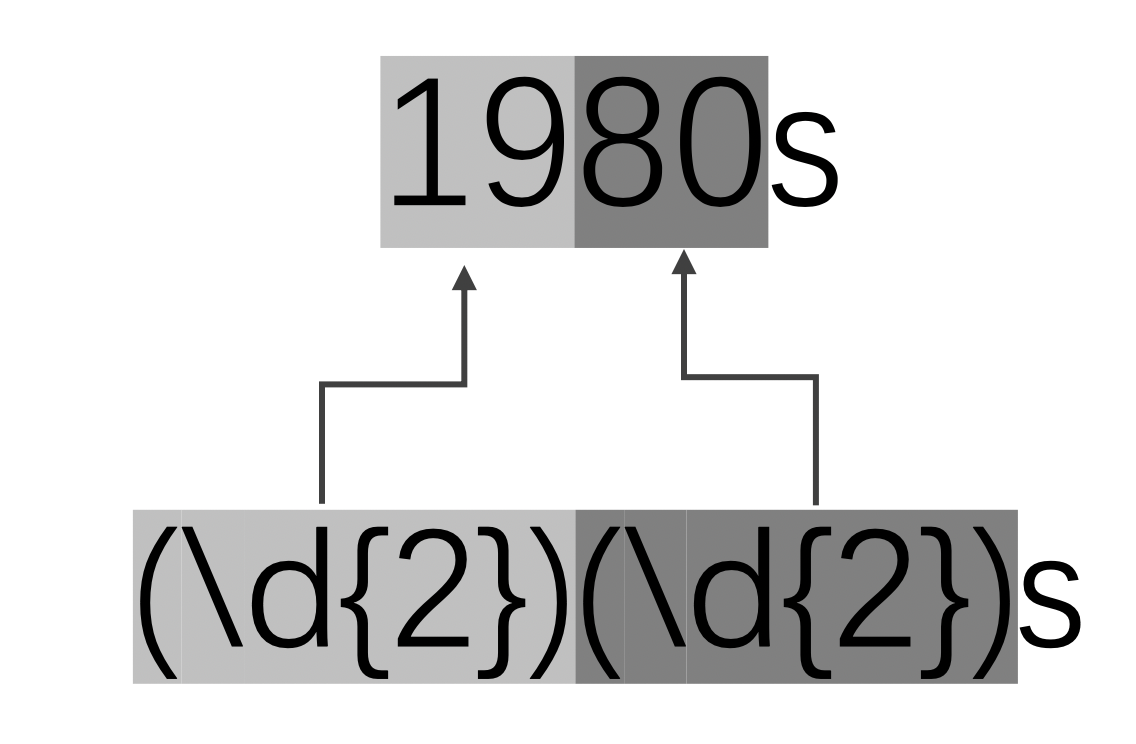#include <fstream>
#include <iostream>
#include <regex>

using namespace std;

int main() {
regex word_regex(R"((\d{2})(\d{2})s)"); // ①

ifstream file("./content.txt");
string line;
while(getline(file, line)) {
auto iter_begin = sregex_iterator(line.begin(),
line.end(),
word_regex);
auto iter_end = sregex_iterator();
for (auto iter = iter_begin; iter != iter_end; iter++) {
cout << "Match content: " << iter->str(0) << ", "; // ②
cout << "group Size: " << iter->size() << endl;  // ③

cout << "Century: " << iter->str(1) << ", "; // ④
cout << "length: " << iter->length(1) << ", ";
cout << "position: " << iter->position(1) << endl;

auto year = (*iter); // ⑤
cout << "Year: " << year.str() << ", ";
cout << "length: " << year.length() << ", ";
cout << "position: " << iter->position(2) << endl;

cout << endl;
}
}

return 0;
}


1. 这个正则表达式请注意其中的圆括号
2. 先打印匹配的字符串整体
3. 所有的分组数量，应该是 2 + 1 = 3
4. 打印出世纪的部分
5. 获取编号2的分组，其类型是sub_match

Match content: 1950s, group Size: 3
Century: 19, length: 2, position: 25
Year: 50, length: 2, position: 27

Match content: 1980s, group Size: 3
Century: 19, length: 2, position: 277
Year: 80, length: 2, position: 279


# 稍微深入一点的内容

## 量词的占有欲

regex content_regex("\"(.+)\"");

• 两边的双引号通过反斜杠转义
• 待捕获的内容通过圆括号形成分组
• 双引号中可以是任意内容，因此使用.+

"find" or "find and replace"


• "find"
• "find and replace"
• "find" or "find and replace"

"find"
"find and replace"


• 匹配优先量词：*+?{num, num}
• 忽略优先量词: *?+???{num, num}?

## 锚点

• 行/字符串的起始位置：^，行/字符串的结束位置：\$
• 单词边界：\b
• 环视 ，见下文

• 正则表达式^\d+在字符串"123abc"中能找到匹配，在字符串"abc123"却找不到。
• 正则表达式some\b在字符串"some birds"中能找到匹配，在字符串"sometimes wonderful"中却找不到。

#include <iostream>
#include <regex>

using namespace std;

void findIn(const char* content, const char* reg_ex) {
cout << "Search '" << reg_ex << "' in '" << content << "': ";
smatch match;
string s(content);
regex reg(reg_ex);
if(regex_search(s, match, reg)) {
cout << match << endl;
} else {
cout << "NOTHING" << endl;
}
}

int main() {
findIn("123abc", "^\\d+");
findIn("abc123", "^\\d+");
cout << endl;
findIn("some birds", "some\\b");
findIn("sometimes wonderful", "some\\b");

return 0;
}


Search '^\d+' in '123abc': 123
Search '^\d+' in 'abc123': NOTHING

Search 'some\b' in 'some birds': some
Search 'some\b' in 'sometimes wonderful': NOTHING


## 环视

1. 匹配出所有sometimes中的前四个字符“some”
2. 匹配出所有的单词some，但是要排除掉“some birds”中的“some”

C++中的环视只支持顺序环视，不支持逆序环视。

#include <iostream>
#include <regex>

using namespace std;

void isMatch(const char* content, const char* reg_ex) {
cout << "Is '" << reg_ex << "' match '" << content << "': ";
smatch match;
string s(content);
regex reg(reg_ex);
if(regex_search(s, match, reg)) {
cout << "YES" << endl;
} else {
cout << "NO" << endl;
}
}

int main() {
isMatch("sometimes", "(?=sometimes)some");
isMatch("something", "(?=sometimes)some");

cout << endl;
isMatch("some eggs", "(?!some birds)some");
isMatch("some birds", "(?!some birds)some");

return 0;
}


Is 'sometimes' match '(?=sometimes)some': YES
Is 'something' match '(?=sometimes)some': NO

Is 'some eggs' match '(?!some birds)some': YES
Is 'some birds' match '(?!some birds)some': NO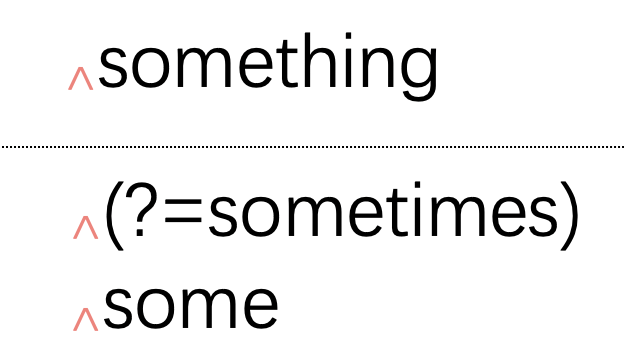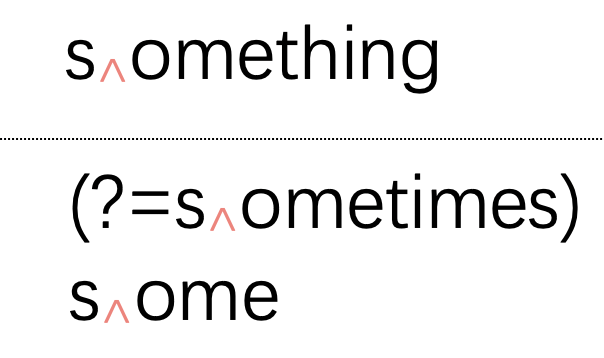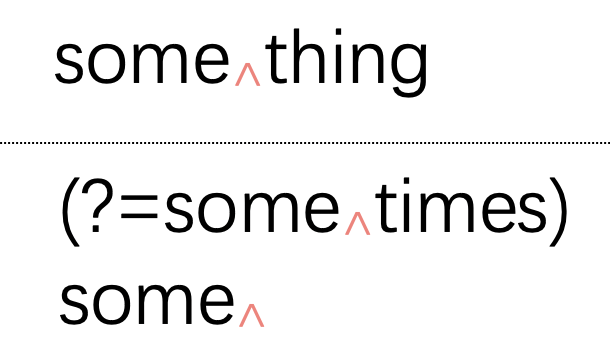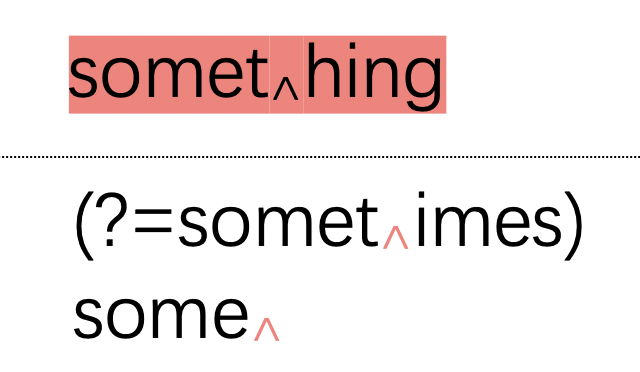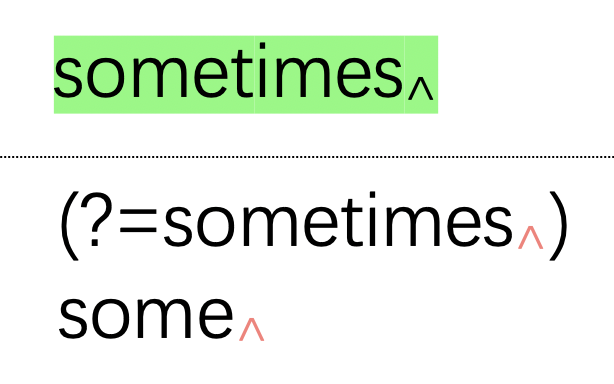Contents